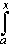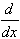#Interactive Real Analysis

Next | Previous | Glossary | Map

## 7.1. Riemann Integral

### Examples 7.1.21(a):

Define a function F(x) =t2 sin(t) dt for x in the interval [a, a + 10].
1. Find F(a)
2. Find F'(x)
3. Find F''(x)
4. Find all critical points of F(x) in [a, a + 10]
1. Since F(x) =t2 sin(t) dt the value F(a) is an integral from a to a. But such an integral is 0 regardless of the integrand. Therefore F(a) = 0.

2. The second part is a direct application of the (second) Fundamental Theorem of Calculus:t2 sin(t) dt = x2 sin(x)

3. Since we have computed the first derivative already, it is easy to compute the second derivative:

F''(x) =F'(x) =x2 sin(x) = 2x sin(x) + x2 cos(x)
4. To find the critical points of F we need to find the points where F is not differentiable or where F'(x) = 0. We know that F is differentiable on any closed interval so that the critical points are those where
F'(x) = x 2 sin(x) = 0
Therefore the critical points are x = 0 and x = k, k=1, 2, ..., or better those points k = 0, 1, ... that are inside the interval [a, a+10].
Next | Previous | Glossary | Map